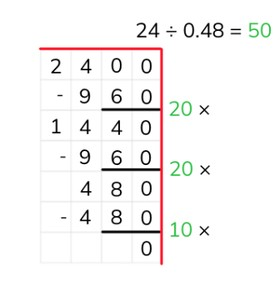Partial quotients division with decimal numbers

# Partial quotients division with decimal numbers

Students learn partial quotients division with decimal numbers.8,000 schools use Gynzy92,000 teachers use Gynzy1,600,000 students use Gynzy

## General

The students learn partial quotients division with a decimal number with 1 or 2 decimal places.

## Standards

CCSS.Math.Content.5.NBT.B.7

## Learning objective

Students will be able to use partial quotients to divide with a decimal number.

## Introduction

On the interactive whiteboard there are a few problems, for which a child tells what the answer is. The students must solve the problem using partial quotients to determine whether each of the children's answers is correct or not.

## Instruction

Explain that with partial quotients division you break the problem up into pieces and then solve it in this way. You write down the problem and put a line underneath it. Next you can use a helping row from the table of the number that you are dividing by. You don't need to include every problem in this row. You can also double or halve problems. First explain how you solve a problem using partial quotients, for which you divide a decimal number by a whole number. Solve the problem step by step by seeing how many times 6 fits in 10.8. First you solve with the number in front of the decimal point. When you can no longer divide, you add the numbers together and put a decimal point after the answer. In the example you can only divide the number 1 time by 6, so you write: 1. ... Next you imagine the decimal point away and solve the rest of the problem. In this case you can still divide the 8 by 6, so after the 1. you put the 8. Have the students practice a few problems using partial quotients. Have them write down their calculations on graphing paper, and they can also include a helping row. After this you explain how you solve a problem using partial quotients, for which you need to divide by a decimal number. Explain that you need to make the decimal number a whole number. Next you can use partial quotients division to solve the problem. Add all of the numbers together to know what the answer is (20 + 20 + 10). Have the students practice with a few problems, for which the divisor is a decimal number.

Check whether the students can use partial quotients to divide through the following questions/assignment:
- Why is it useful to be able to divide using partial quotients?
- What aid can you use when dividing using partial quotients?
- Solve this problem using partial quotients division: 16.2 ÷ 2,7

## Quiz

The students test their understanding of partial quotients division with decimal numbers through ten exercises. In some of the exercises, the students must divide a decimal number. In others, they must divide by a decimal number. And in other exercises, both the dividend and the divisor are decimal numbers.

## Closing

You discuss once again with the students how it is important to be able to divide decimal numbers using partial quotients, because that is an easier way to solve problems. Check if the students know that with partial quotients division you solve pieces of the problem in steps and that they can use a helping row to do so. On the interactive whiteboard there are a few problems for the students to solve using partial quotients division.

## Teaching tips

The students first practice with partial quotients division, for which the first number of the problem is a decimal number. Next the divide by a decimal number. Finally they solve a problem using partial quotients, for which both the first and the second number of the problem are decimal numbers.

## Instruction materials

Graphing paper

### The online teaching platform for interactive whiteboards and displays in schools

• Save time building lessons

• Manage the classroom more efficiently

• Increase student engagement